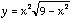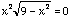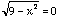Name: Sally Who is asking: Student Question: I am looking for any intecepts. How do I solve: y=x^2 square root of (9-x^2) Hi Sally The x-intercepts occur where y=0 and the y-intercepts where x=0. Your expression isand hence substituting x=0 gives you y=0. Thus the only y-intercept is at (0,0). To find the x-intercepts set y=0 to getThe left side of this expression is the product of two numbers, x2 and the square root of (9-x2). If this product is 0 then either x2 is 0 or the square root of (9-x2) is 0. Hence the x-interceps occur at points where either x2=0 orCheers Harley

Go to Math Central